# LMN – Digital Electronics

See Last Minute Notes on all subjects here.
We will discuss the important key points useful for GATE exams in summarized form. For details you may refer this.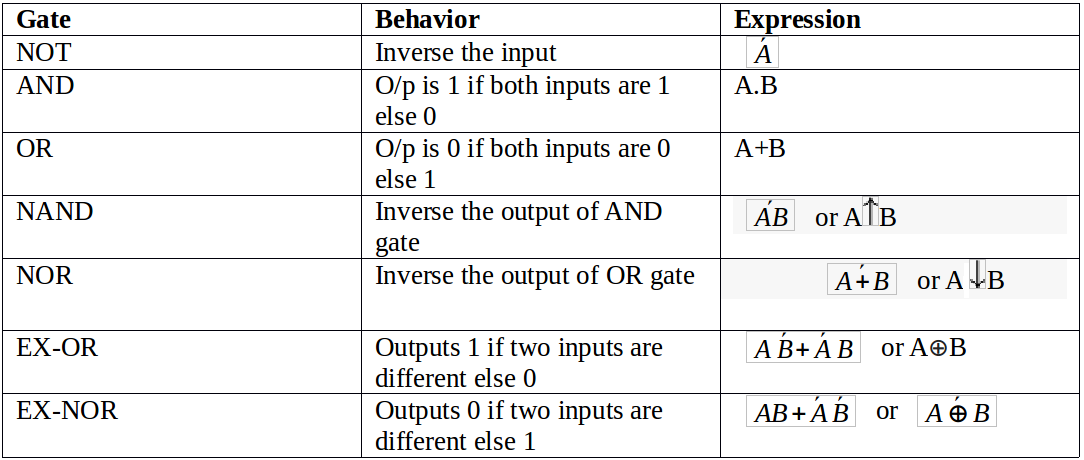• Note: NAND and NOR gates are called UNIVERSAL GATES because all other gates can be constructed from any of them.

K-map example of 3 variables: : F(A,B,C)=π(0,3,6,7)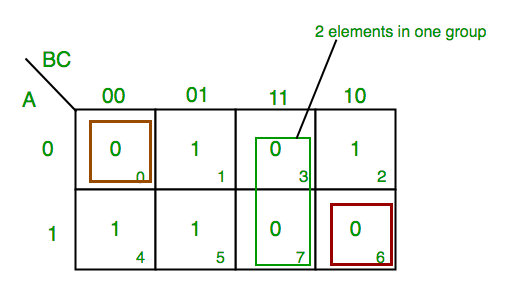From red group we find terms

`A    B      C’`

Taking complement of these two

`A’     B’     C`

Now sum up them
(A’ + B’ + C)
From green group we find terms

`B         C`

Taking complement of these two terms

`B’         C’`

Now sum up them
(B’+C’)
From brown group we find terms

`A’ B’ C’`

Taking complement of these two

`A B C`

Now sum up them
(A + B + C)
We will take product of these three terms :Final expression (A’ + B’ + C) (B’ + C’) (A + B + C)

Number of NAND or NOR gate required to implement other GATE: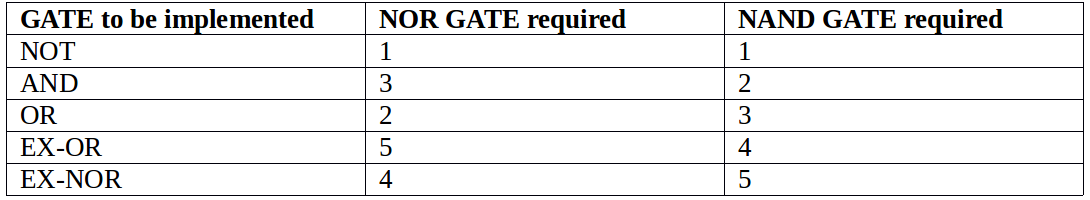Combinational circuits: In combinational circuits, output depends on present input only; it does not require any feedback and memory.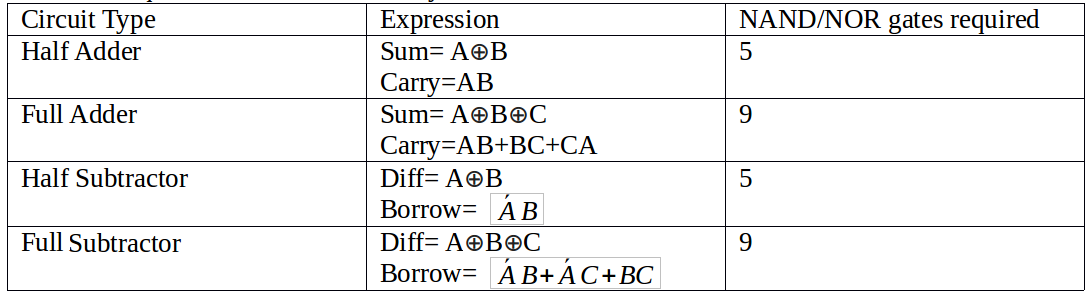Multiplexer : It selects the input from one of many input lines and sends it single output line. The input line chosen from output is based upon set of selection lines.For 2n input lines, there will be n select lines and 1 output lines.

Note: No. of mux required to implement n x 1 mux using m x 1 mux is ceil(n-1 / m-1)A⊕B.
No. of mux required to implement 16 x 1 mux using 4 x 1 mux is ceil(15/3)=5.

Demultiplexer: It selects the input from one input line and sends it to one out of many output lines. The output line chosen is based upon set of selection lines. For 1 input lines, there will be n select lines and 2n output lines.

Note: No. of mux required to implement 1 x n mux using 1 x m mux is ceil(n-1 / m-1)A⊕B.
No. of mux required to implement 1 x 16 mux using 1 x 4 mux is ceil(15/3)=5.

Encoder: For 2n input lines, it has n output. It can be used to convert octal or hexadecimal to binary data.

Decoder: For n input lines, it has either 2n outputs or less then that. It can be used to convert binary data to other codes like octal or hexadecimal.

Code Converter: Code converters are used to convert one type of code to others.

• BCD to Excess-3: It will add 0011(value 3) to binary code.
Function:

4 bit Input(ABCD) with A as MSB and 4 bit output(WXYZ) with W as MSB, O/P is
Z=D’
Y=CD + C’D’
X=B’D + B’C + BC’D’
W=A + BC + BD
• Binary to Gray Converter
Function:

4 bit Input(B3B2B1B0) with B3 as MSB and 4 bit output(G3G2G1G0) with G3 as MSB, O/P is
G3=B3
G2=B3⊕ B2
G1=B2⊕ B1
G0=B1⊕ B0
• Gray to Binary Converter
Function:

4 bit Input(G3G2G1G0) with G3 as MSB and 4 bit output(B3B2B1B0) with B3 as MSB, O/P is
B3=G3
B2=B3⊕G2
B1=B2⊕G1
B0=B1⊕G0

Flip-Flops: Flip-Flop are sequential circuits where O/P depends on present input as well as previous output.

S-R Flip-Flops: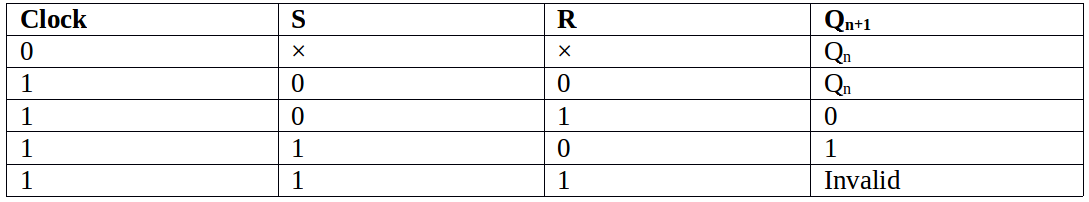Characteristics equation: Qn = S + R̄ Qn

J-K Flip-Flops: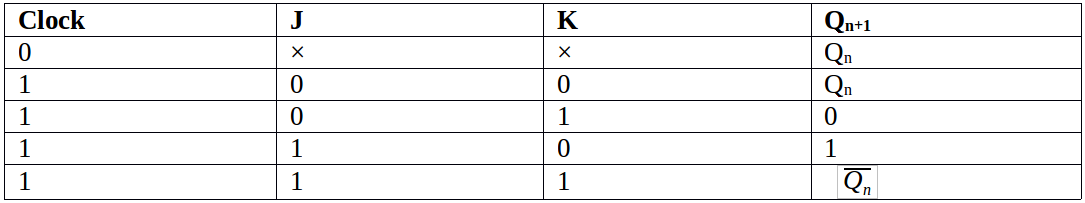Characteristics equation: Qn = J Q̄n + R̄ Qn

D Flip-Flops: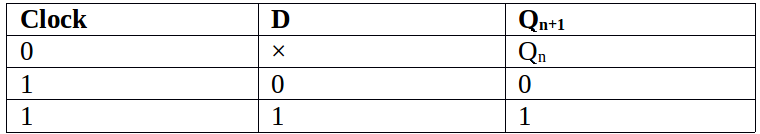Characteristics equation: Qn+1 = D

T Flip-Flops: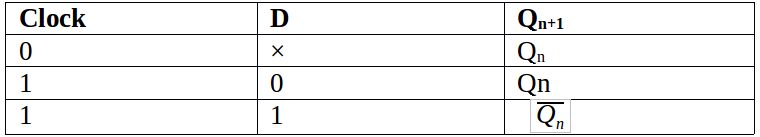Characteristics equation: Qn+1 = T Q̄n + T̄ Qn

Counters is a device which stores (and sometimes displays) the number of times a particular event or process has occurred, often in relationship to a clock signal.

Counters are basically divided into two types:

Asynchronous counter: In asynchronous counter we don’t use universal clock, only first flip flop is driven by main clock and the clock input of rest of the following counters is driven by output of previous flip flops.

Synchronous counter: Unlike the asynchronous counter, synchronous counter has one global clock which drives each flip flop so output changes in parallel. The one advantage of synchronous counter over asynchronous counter is, it can operate on higher frequency than asynchronous counter as it does not have cumulative delay because of same clock is given to each flip flop.

Important point: Number of flip flops used in counter are always greater than equal to (log2 n) where n=number of states in counter.